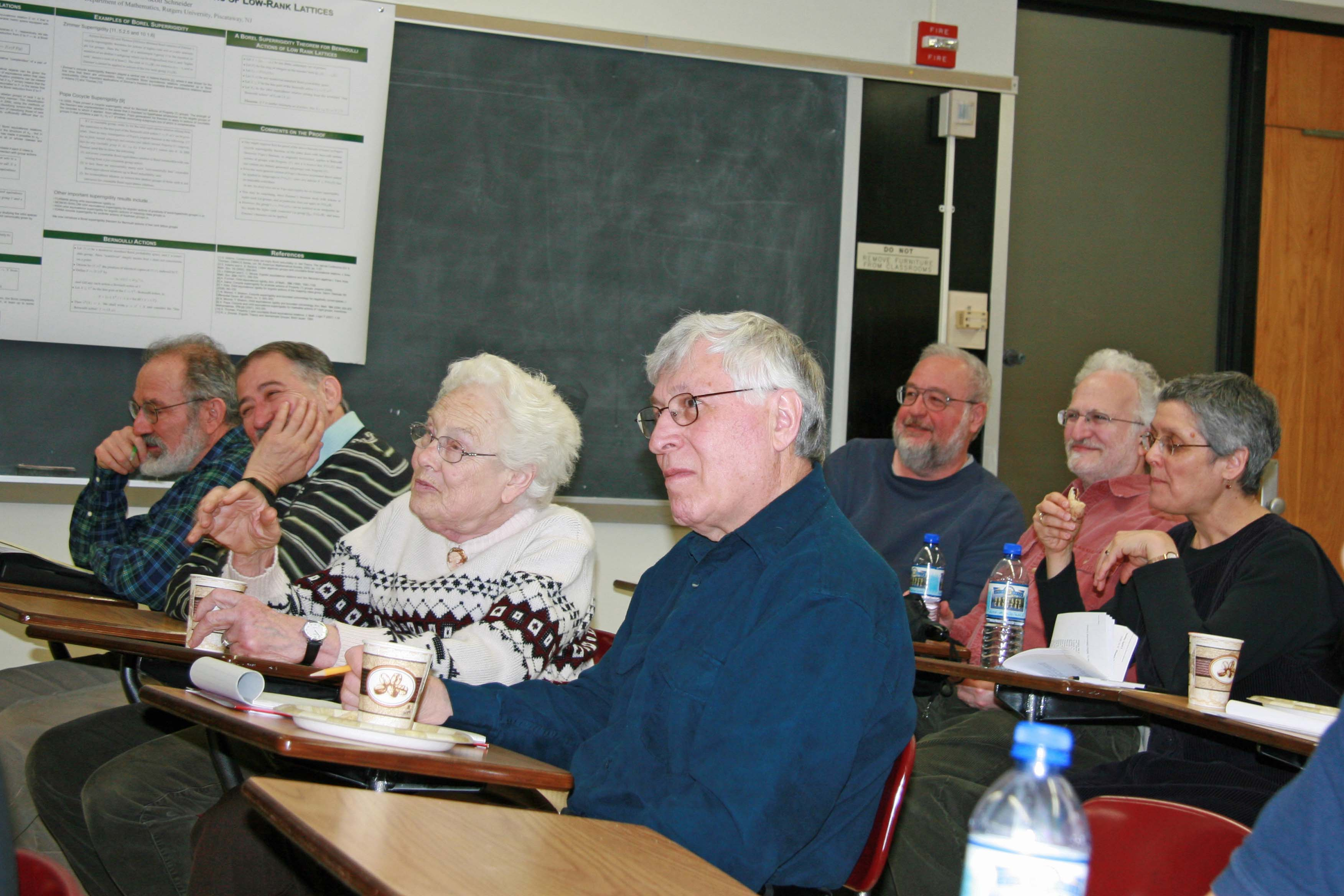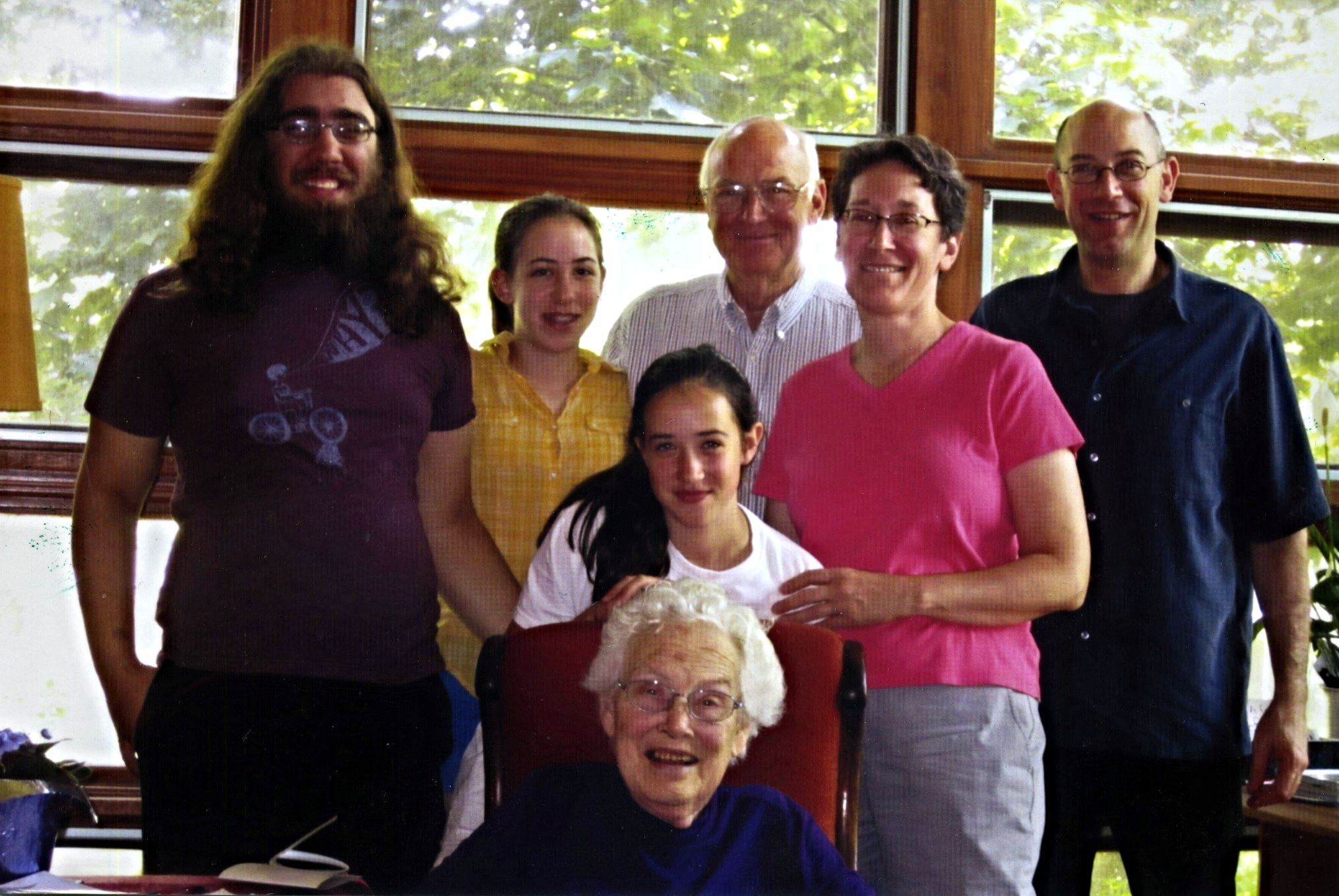Celebratio Mathematica

Mary Ellen Rudin

A Tribute to Mary Ellen Rudin

by Kenneth Kunen and Arnold Miller

There is an ex­cel­lent bio­graph­ic­al in­ter­view of Mary El­len pub­lished in [e2]. Also, there is an en­tire volume [e3] de­voted to art­icles de­scrib­ing her math­em­at­ics. All quo­ta­tions be­low are taken from [e3]. Mary El­len Rud­in was one of the lead­ing to­po­lo­gists of our time. Be­sides solv­ing a num­ber of well-known out­stand­ing open prob­lems, she was a pi­on­eer in the use of set-the­or­et­ic tools. She was one of the first to ap­ply the in­de­pend­ence meth­ods of Co­hen and oth­ers to pro­duce in­de­pend­ence res­ults in to­po­logy. She did not do for­cing ar­gu­ments her­self, but many of her pa­pers make use of set-the­or­et­ic state­ments, such as Mar­tin’s Ax­iom, Suslin’s Hy­po­thes­is, and $\diamond$, that oth­er re­search­ers have shown to be true in some mod­els of set the­ory and not in oth­ers.

In her thes­is  she gave an ex­ample of a non­sep­ar­able Moore space that sat­is­fies the count­able chain con­di­tion. She pub­lished the res­ults of her thes­is in three pa­pers in the Duke Math Journ­al , , . To quote Steve Wat­son, “This cycle rep­res­ents one of the greatest ac­com­plish­ments in set-the­or­et­ic to­po­logy. However the math­em­at­ics in these pa­pers is of such depth that, even forty years later, they re­main im­pen­et­rable to all but the most di­li­gent and pa­tient of read­ers.”

In  she used a Suslin tree to con­struct a Dowker space. The ex­ist­ence of a Suslin tree is con­sist­ent with ZFC but not prov­able from ZFC. In   she con­struc­ted a Dowker space just in ZFC. This space has car­din­al­ity $(\mathfrak{N}_\omega )^{\mathfrak{N}_0}$, where­as the Suslin tree yields a Dowker space of size only $\mathfrak{N}_1$. Also, in 1976  she showed that CH yields a Dowker space of size $\mathfrak{N}_1$. It is still an open ques­tion wheth­er one can prove in ZFC that there is a Dowker space of size $\mathfrak{N}_1$. There is one of size $\mathfrak{N}_{\omega+1}$ by Ko­j­man and She­lah [e4]. Her work on Dowker spaces led to an in­vited ad­dress at the In­ter­na­tion­al Con­gress of Math­em­aticians in 1974. It also led to her in­terest in the box to­po­logy, be­cause her Dowker space of size $(\mathfrak{N}_{\omega})^{\mathfrak{N}_0}$ is a spe­cial kind of box product.

Mary El­len is fam­ous for her work on box products. If $X_n$ (for $n \in \omega$) are to­po­lo­gic­al spaces, then $\square_n X_n$ de­notes the product of the spaces $\prod_n X_n$ us­ing the box to­po­logy; a base for this to­po­logy is giv­en by all sets $\prod_n U_n$, where each $U_n$ is open in $X_n$. The more well-known Ty­chon­ov to­po­logy, used to prove the Ty­chon­ov The­or­em (1935), re­quires that $U_n = X_n$ for all but fi­nitely many $n$. Mary El­len was the first per­son to prove any­thing non­trivi­al about box products. In  she showed that, as­sum­ing $\mathrm{CH}$, $\square_n X_n$ is nor­mal, and in fact para­com­pact, whenev­er all the $X_n$ are com­pact met­ric spaces. Also, her 1974 pa­per  shows that $\square_n X_n$ is para­com­pact whenev­er all the $X_n$ are suc­cessor or­din­als; this eas­ily gen­er­al­izes to the case where the $X_n$ are com­pact scattered spaces (see [e1]). It was later shown by Eric van Douwen, in ZFC, that there are al­ways com­pact Haus­dorff $X_n$ for which $\square_n X_n$ is not nor­mal. The ques­tion of which of Mary El­len’s pos­it­ive res­ults can be proved in $\mathrm{ZFC}$ re­mains an out­stand­ing un­solved prob­lem in to­po­logy. Even the case where all $X_n = \omega + 1$ re­mains open, al­though this case (or when all $X_n$ are com­pact met­ric) does fol­low from Mar­tin’s Ax­iom.

Mary El­len is also fam­ous for her work on $\beta \mathbb{N}$, the space of ul­tra­fil­ters on the nat­ur­al num­bers, start­ing in 1966. She was coin­vent­or of two well-known par­tial or­ders on this space: the Rud­in–Keisler or­der and the Rud­in–Fro­lik or­der. The ba­sic prop­er­ties of these are giv­en in her 1971 pa­per , al­though their his­tor­ic roots go back some­what earli­er.

The Rud­in–Fro­lik or­der led to the first proof in ZFC that the space of non­prin­cip­al ul­tra­fil­ters, $\mathbb{N}^\ast = \beta\mathbb{N} \backslash \mathbb{N}$, is not ho­mo­gen­eous. Un­der CH this was already known by a res­ult of Wal­ter Rud­in (1956), who proved from CH that there is a P-point $\mathcal{U} \in \mathbb{N}^\ast$ (that is, $\mathcal{U}$ is in the in­teri­or of every $G_\delta$ set con­tain­ing $\mathcal{U}$). Non­homo­gen­eity fol­lows be­cause every in­fin­ite com­pactum also has a non-P-point $\mathcal{V}$, and no homeo­morph­ism of $\mathbb{N}^\ast$ can move this $\mathcal{V}$ to Wal­ter’s $\mathcal{U}$. It was shown much later (She­lah, in the 1970s) that one can­not prove in ZFC that these P-points ex­ist.

For the Rud­in–Fro­lik or­der, for $\mathcal{U},\mathcal{V}\in \mathbb{N}^\ast$, say that $U < _{\mathrm{RF}}\mathcal{V}$ iff $\mathcal{V}$ is a $\mathcal{U}$-lim­it of some dis­crete $\omega$-se­quence of points in $\mathbb{N}^\ast$. One can show that this is a par­tial or­der; also, if $\mathcal{U} < _{\mathrm{RF}}\mathcal{V}$, then no homeo­morph­ism of $\mathbb{N}^\ast$ can move $\mathcal{V}$ to $\mathcal{U}$, prov­ing non­homo­gen­eity.At the Kunen Fest 2009: (left to right/front row, back row) Ken Kunen, István Juhász, Mary Ellen, Jerry Keisler, Arnold Miller, Franklin Tall, Judy Roitman.

The Rud­in–Keisler or­der comes nat­ur­ally out of the no­tion of in­duced meas­ure. If $\mathcal{U}, \mathcal{V}$ are ul­tra­fil­ters on any set $I$, we say that $\mathcal{U} \leq_{\mathrm{RK}}\mathcal{V}$ iff there is a map $f : I \rightarrow I$ that in­duces the meas­ure $\mathcal{U}$ from $\mathcal{V}$; that is, $\mathcal{U} = \{X \subseteq I : f^{-1} (X) \in \mathcal{V} \} .$ When $U, \mathcal{V} \in \mathbb{N}^\ast$, $\mathcal{U} < _{\mathrm{RF}} \mathcal{V}$ im­plies that $U \leq_{\mathrm{RK}} \mathcal{V}$ and $\mathcal{V} \nleq_{\mathrm{RK}}\mathcal{U}$. This or­der is of im­port­ance in mod­el the­ory, in the the­ory of ul­traproducts, since $\mathcal{U} \leq_{\mathrm{RK}} \mathcal{V}$ yields a nat­ur­al ele­ment­ary em­bed­ding from $\prod_i \mathfrak{A}_i /\mathcal{U}$ in­to $\prod_i \mathfrak{A}_i /\mathcal{V}$. It is also im­port­ant in the com­bin­at­or­ics of ul­tra­fil­ters, since the Rud­in–Keisler min­im­al ele­ments of $\mathbb{N}^\ast$ are pre­cisely the Ram­sey ul­tra­fil­ters; such min­im­al ele­ments ex­ist un­der CH but not in ZFC. It is true, but not com­pletely trivi­al, that neither of these two or­ders is a total or­der; that is, in ZFC there are $\mathcal{U}, \mathcal{V} \in \mathbb{N}^\ast$ such that $\mathcal{U} \nleq_{\mathrm{RK}} \mathcal{V}$ and $\mathcal{V} \nleq_{\mathrm{RK}} \mathcal{U}$ (and hence also $\mathcal{U} \nless_{\mathrm{RF}} \mathcal{V}$ and $\mathcal{V} \nless_{\mathrm{RF}} \mathcal{U}$). The pa­per  (1979) of Mary El­len and Sahar­on She­lah gives the strongest pos­sible res­ult: for each in­fin­ite $\kappa$, there is a fam­ily of $2^{2^\kappa}$ ul­tra­fil­ters on $\kappa$ that are pair­wise in­com­par­able in the Rud­in–Keisler or­der­ing. Mary El­len worked ex­tens­ively on the ques­tion of $S$- and $L$-spaces. She pro­duced the first $S$-space (a hered­it­ar­ily sep­ar­able space that is not hered­it­ar­ily Lindelöf) as­sum­ing the ex­ist­ence of a Suslin tree in 1972 . Her Dowker space con­struc­ted from CH (see above) is also an $S$-space. Her 1975 mono­graph  de­votes an en­tire chapter to $S$- and $L$-spaces. To quote Stevo To­dorčević, “The terms ‘$S$-space’ and ‘$L$-space,’ which are pre­dom­in­ant in most of the lit­er­at­ure on this sub­ject, are also first found in these lec­tures. This shows a great in­flu­ence not only of  but also of M. E. Rud­in’s per­son­al­ity on the gen­er­a­tion of math­em­aticians work­ing in this area, since it is rather un­usu­al in math­em­at­ics to talk about cer­tain state­ments in terms of their counter­examples.”

In 1999, al­most a dec­ade after her re­tire­ment, Mary El­len settled a long-stand­ing con­jec­ture in set-the­or­et­ic to­po­logy by show­ing that every mono­ton­ic­ally nor­mal com­pact space is the con­tinu­ous im­age of a lin­early ordered com­pact space. This pa­per  was the fi­nal one in a series of five which gradu­ally settled more and more spe­cial cases of the fi­nal res­ult. The con­struc­tion of the lin­early ordered com­pact space is ex­traordin­ar­ily com­plex, and to this date there is no sim­pler proof known.At the time of Walter’s memorial gathering in 2010: Mary Ellen with grandson Deniz, granddaughter Sofie, brother Joe Estill, daughter Eleanor, son Charlie, and granddaughter Natalie. (Photo courtesy of Scott Gaff.)

Mary El­len was by con­sensus a dom­in­ant fig­ure in gen­er­al to­po­logy. Her res­ults are dif­fi­cult, deep, ori­gin­al, and im­port­ant. The con­nec­tions she found between to­po­logy and lo­gic at­trac­ted many set-the­or­ists and lo­gi­cians to to­po­logy. The best gen­er­al to­po­lo­gists and set-the­or­ists in the world passed reg­u­larly through Madis­on to vis­it her and work with her and her stu­dents and col­leagues.

She had eight­een PhD stu­dents, many of whom went on to have ster­ling ca­reers of their own. To quote one of them, Mi­chael Star­bird, “From the per­spect­ive of a gradu­ate stu­dent and col­lab­or­at­or, her most re­mark­able fea­ture is the flood of ideas that is con­stantly burst­ing from her…. It is easy to use the Mary El­len Rud­in mod­el to be­come a great ad­visor. The first step is to have an end­less num­ber of great ideas. Then merely give them totally gen­er­ously to your stu­dents to de­vel­op and learn from. It is really quite simple. For Mary El­len Rud­in.”

Works

 M. E. Es­till: Con­cern­ing ab­stract spaces. Ph.D. thesis, Uni­versity of Texas at Aus­tin, 1949. Ad­vised by R. L. Moore. A con­densed ver­sion was pub­lished in Duke Math. J. 17:4 (1950). MR 2937954 phdthesis

 M. E. Es­till: “Con­cern­ing ab­stract spaces,” Duke Math. J. 17 : 4 (1950), pp. 317–​327. A con­densed ver­sion of the au­thor’s PhD thes­is (1949). MR 42686 Zbl 0039.​39303 article

 M. E. Es­till: “Sep­ar­a­tion in non-sep­ar­able spaces,” Duke Math. J. 18 : 3 (1951), pp. 623–​629. MR 42687 Zbl 0044.​19503 article

 M. E. Es­till: “Con­cern­ing a prob­lem of Souslin’s,” Duke Math. J. 19 : 4 (1952), pp. 629–​639. MR 50878 Zbl 0048.​28401 article

 M. E. Rud­in: “Count­able para­com­pact­ness and Souslin’s prob­lem,” Can. J. Math. 7 (February 1955), pp. 543–​547. MR 73155 Zbl 0065.​38002 article

 M. E. Rud­in: “A nor­mal space $X$ for which $X\times I$ is not nor­mal,” Bull. Am. Math. Soc. 77 : 2 (March 1971), pp. 246. An ex­pan­ded ver­sion of this was pub­lished in Fund. Math 73:2 (1971–1972). MR 270328 Zbl 0206.​51601 article

 M. E. Rud­in: “Par­tial or­ders on the types in $\beta N$,” Trans. Am. Math. Soc. 155 : 2 (April 1971), pp. 353–​362. MR 273581 Zbl 0212.​54901 article

 M. E. Rud­in: “A nor­mal space $X$ for which $X\times I$ is not nor­mal,” Fund. Math. 73 : 2 (1971–1972), pp. 179–​186. A brief ini­tial ver­sion was pub­lished in Bull. Am. Math. Soc. 77:2 (1971). MR 293583 Zbl 0224.​54019 article

 M. E. Rud­in: “A nor­mal hered­it­ar­ily sep­ar­able non-Lindelöf space,” Ill. J. Math. 16 : 4 (1972), pp. 621–​626. MR 309062 Zbl 0241.​54013 article

 M. E. Rud­in: “The box product of count­ably many com­pact met­ric spaces,” Gen­er­al To­po­logy Ap­pl. 2 : 4 (December 1972), pp. 293–​298. MR 324619 Zbl 0243.​54015 article

 M. E. Rud­in: “Count­able box products of or­din­als,” Trans. Am. Math. Soc. 192 (1974), pp. 121–​128. MR 340022 Zbl 0289.​02052 article

 M. E. Rud­in: Lec­tures on set the­or­et­ic to­po­logy (Lara­m­ie, WY, 12–16 Au­gust 1974). CBMS Re­gion­al Con­fer­ence Series in Math­em­at­ics 23. Amer­ic­an Math­em­at­ic­al So­ci­ety (Provid­ence, RI), 1975. Re­prin­ted in 1980. MR 367886 Zbl 0318.​54001 book

 I. Juhász, K. Kun­en, and M. E. Rud­in: “Two more hered­it­ar­ily sep­ar­able non-Lindelöf spaces,” Can. J. Math. 28 : 5 (October 1976), pp. 998–​1005. MR 428245 Zbl 0336.​54040 article

 S. She­lah and M. E. Rud­in: “Un­ordered types of ul­tra­fil­ters,” To­po­logy Proc. 3 : 1 (1978), pp. 199–​204. MR 540490 Zbl 0431.​03033 article

 M. E. Rud­in: “Nikiel’s con­jec­ture,” To­po­logy Ap­pl. 116 : 3 (December 2001), pp. 305–​331. MR 1857669 Zbl 0988.​54022 article